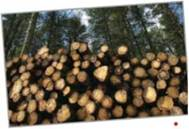Chapter 6.2, Problem 62E

Chapter
Section
Textbook Problem

ForestryThe value of a tract of timber is V ( t ) = 100 , 000 e 0.8 t where t is the time in years, with t = 0 corresponding to 2010.If money earns interest continuously at 10%, then the present value of the timber at any time t is A ( t ) = V ( t ) e − 0.10 t .Find the year in which the timber should be harvested to maximize the present value function.To determine

To calculate: The year in which timber has be harvested in order to maximize the present value function.

Explanation

Given:

The value of a tract of timber is V(t)=100000e0.8t where t is the time in years, with t=0 corresponding to 2010.

If money earns interest continuously at 10%, then the present value of the timber at any time t is,

A(t)=V(t)e0.10t.

Formula used:

ddxuv=udvdx+vdudx

ddx(xn)=nxn1

ddxex=ex

Calculation:

Let us consider the expression, A(t)=V(t)e0.10t.

By putting V(t)=1000000e0.8t in above equation, we get

A(t)=V(t)e0.10t=100000e0.8t×e0.10t

Now, we have to differentiate the function with respect to t to maximize the function and put in equal to 0. Therefore,

dA(t)dt=d(100000e0

Still sussing out bartleby?

Check out a sample textbook solution.

See a sample solution

The Solution to Your Study Problems

Bartleby provides explanations to thousands of textbook problems written by our experts, many with advanced degrees!

Get Started

Evaluate the expression sin Exercises 116. 23

Finite Mathematics and Applied Calculus (MindTap Course List)

For the following set of scores, find the value of each expression: a. X b. (X)2 c. X2 d. (X +3)

Essentials of Statistics for The Behavioral Sciences (MindTap Course List)

Combine the following fractions.

Mathematical Applications for the Management, Life, and Social Sciences